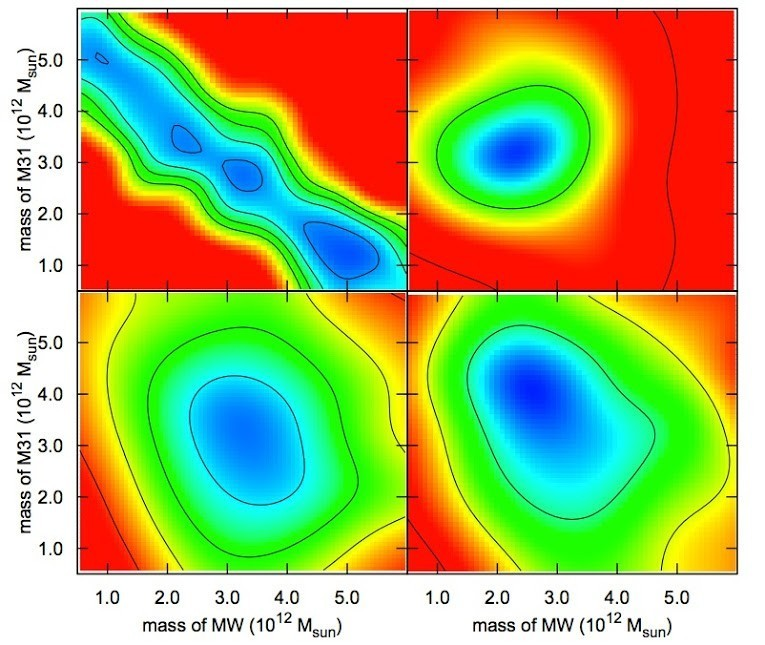# Galactic Scale

In Galaxies by Brian Koberlein2 Comments

How do you weigh a galaxy?

With planets we can measure their distance from the Sun and their orbital speed. By observing their motion in detail we can calculate their mass very precisely. For binary stars we can use a similar method. Observe the size of their mutual orbits and their orbital period, and by Kepler’s laws you can determine their mass. We can use the same method to calculate the mass of the supermassive black hole in the center of our galaxy.

But all of these methods rely on observing the change of an object’s speed over time (its acceleration) either directly or indirectly. For galaxies you can’t really do that. Galaxies aren’t a single object moving in a simple way, but rather a complex system of stars, gas and dust all moving and interacting.

One way that we can calculate the mass of a galaxy is to observe the motion of particular stars in the galaxy. Their accelerations are too small to observe, but by looking at how the speeds of stars closer to the center compare to speeds further from the center we can get an idea of a galaxy’s mass. But because much of a galaxy’s mass is due to dark matter, it is difficult to determine the total mass of a galaxy. We can infer the distribution of dark matter in regions where there are stars, but how do we determine how far beyond the stars the dark matter extends (known as the dark matter halo).

In a recent paper in the Astrophysical Journal, a team looked at a computational approach to determining the mass of galaxies, in particular the masses of the Milky Way and the Andromeda galaxy. Their method was to look at the gravitational attraction between the two galaxies.

While the Milky Way and Andromeda are gravitationally attracting each other, that attraction isn’t very large. Sure, they both have billions of stars worth of mass, but they are also more than 2 million light years apart, and gravitational attraction is weaker at larger distances. So there isn’t a way to measure the acceleration due to gravity. We do, however, have a measure of the speeds of the galaxies relative to each other, and it is this data that the team analyzed.

Basically, the team ran a computer simulation of the two galaxies, along with other members of the local group, using a method known as the numerical action method. This method assumes a mass for both galaxies, then calculates their velocities due to gravity following the principle of least action (which makes the velocities easier to calculate). By calculating the galactic velocities for a range of masses, you can compare the result with the actual observed motion. The better the statistical match, the more likely your assumed masses are the actual masses.

You can see the computational results in the figure above. Each of the four images starts with different data from the local group, and the more blue regions are closer matches to observation. By combining these different results, the best match is a mass of 2.5 trillion solar masses for the Milky Way, and 3.5 trillion solar masses for Andromeda (give or take a trillion solar masses with 95% confidence).

So it turns out we can weigh a galaxy, it just takes some computational physics to do it.

1.2.